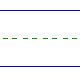Name: ______________________________________________ Date: _____________

Instructions: Find the sum.

 8 + 7 =3 + 9 =5 + 5 =5 + 7 =7 + 7 =8 + 5 =5 + 8 =3 + 7 =2 + 8 =5 + 9 =8 + 3 =9 + 6 =4 + 8 =9 + 4 =9 + 2 =7 + 5 =9 + 3 =9 + 9 =